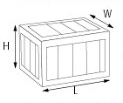## VOLUMETIC WEIGHT

 Volumetric Weight Calculation : - When dimensional weight exceeds actual weight, shipments will be charged according to the IATA volumetric standard.

Chargeable Weight :

As per the regulations of the International Air Transport Association, air carriers charge either by weight or volume, whichever is greater. This is known as chargeable weight. Compare your shipments actual weight versus its volumetric weight and see which value is greater. If the dimensional weight is greater in value than the actual weight, transportation charges will be based on the dimensional weight value. We will always charge the greater of the two amounts.

Domestic Package Formula :Dimensional weight  (lbs) = L X W X H  -------------         166 L = Length in inches W = Width in inches H = Height in inches

International Package Formula :Dimensional weight  (lbs) = L X W X H  -------------         139 L = Length in inches W = Width in inches H = Height in inches Dimensional weight  (Kg) = L X W X H  -------------        5000 L = Length in centimeters W = Width in centimeters H = Height in centimeters
 Measures Conversion : - Length Multiply by Inches to Centimetres 2.54 Centimetres to Inches 0.39 Inches to Millimetres 25.40 Millimetres to Inches 0.04 Feet to Metres 0.31 Metres to Feet 3.28 Yards to Metres 0.91 Metres to Yards 1.09 Miles to Kilometres 1.61 Kilometres to Miles 0.62 Area Multiply by Square Inches to Square Centimetres 6.451 Square Centimetres to Square Inches 0.15 Square Feet to Square Metres 0.09 Square Metres to Square Feet 10.76 Square Yards to Square Metres 0.84 Square Metres to Square Yards 1.20 Square Miles to Square Kilometres 2.59 Square Kilometres to Square Miles 0.39 Acres to Hectares 0.40 Hectares to Acres 2.47 Volume Multiply by Cubic Inches to Cubic Centimetres 16.39 Cubic Centimetres to Cubic Inches 0.06 Cubic Feet to Cubic Metres 0.03 Cubic Metres to Cubic Feet 35.32 Cubic Yards to Cubic Metres 0.76 Cubic Metres to Cubic Yards 1.31 Cubic Inches to Litres 0.02 Litres to Cubic Inches 61.03 Gallons to Litres 4.55 Litres to Gallons 0.22 US Gallons to Litres 3.79 Litres to US Gallons 0.26 Fluid Ounces to Cubic Millilitres 30.77 Cubic Millilitres to Fluid Ounces 0.03 Weight Multiply by Ounces to Grams 28.35 Grams to Ounces 0.04 Pounds to Kilograms 0.45 Kilograms to Pounds 2.21 Long Tons to Tonnes 1.02 Tonnes to Long Tons 0.98 Short Tons to Tonnes 0.91 Tonnes to Short Tons 1.10